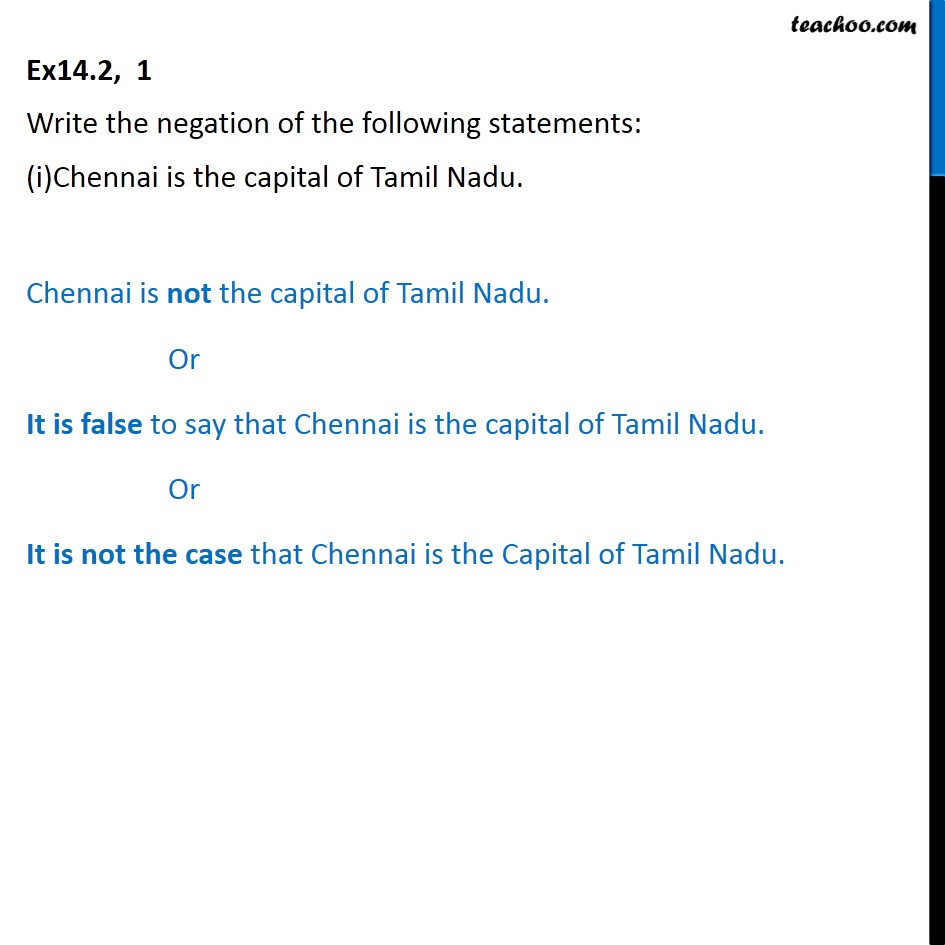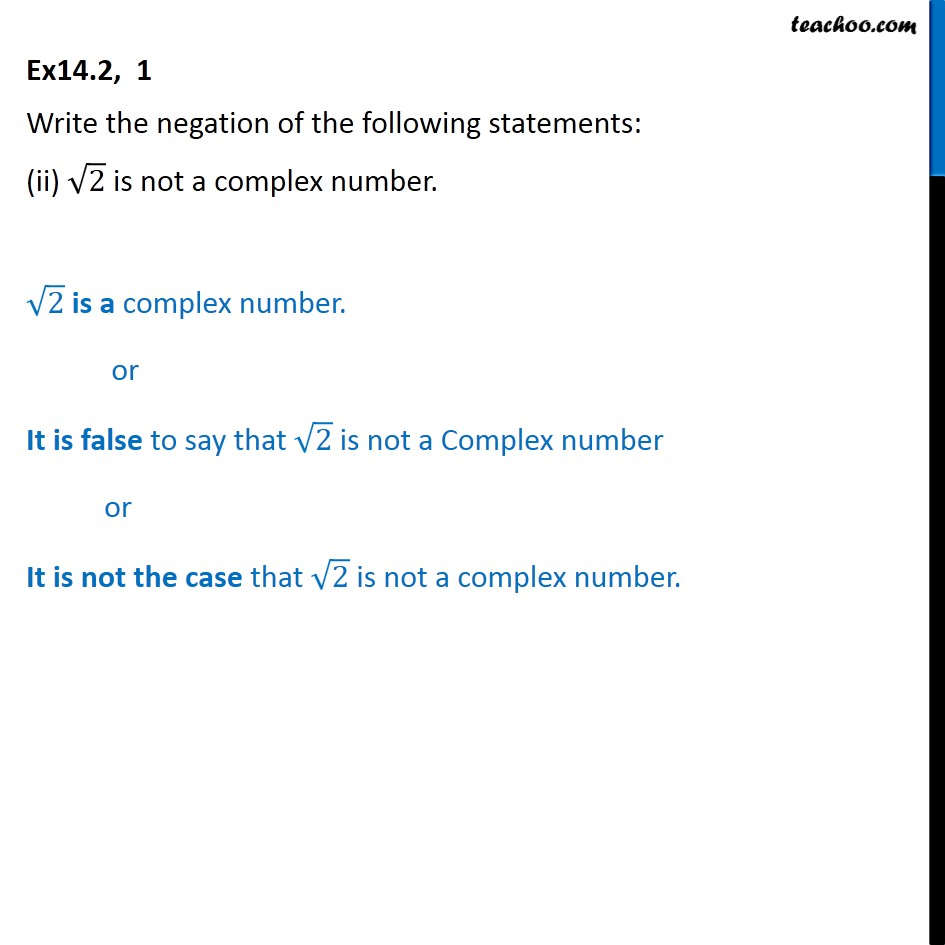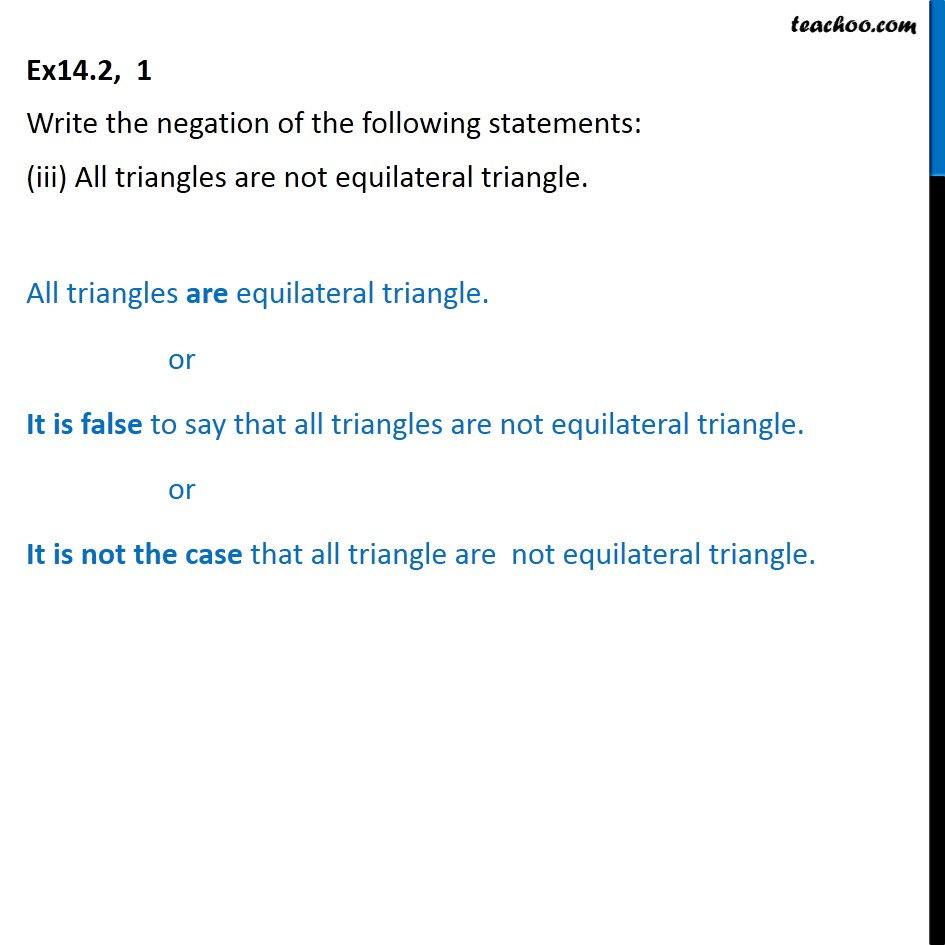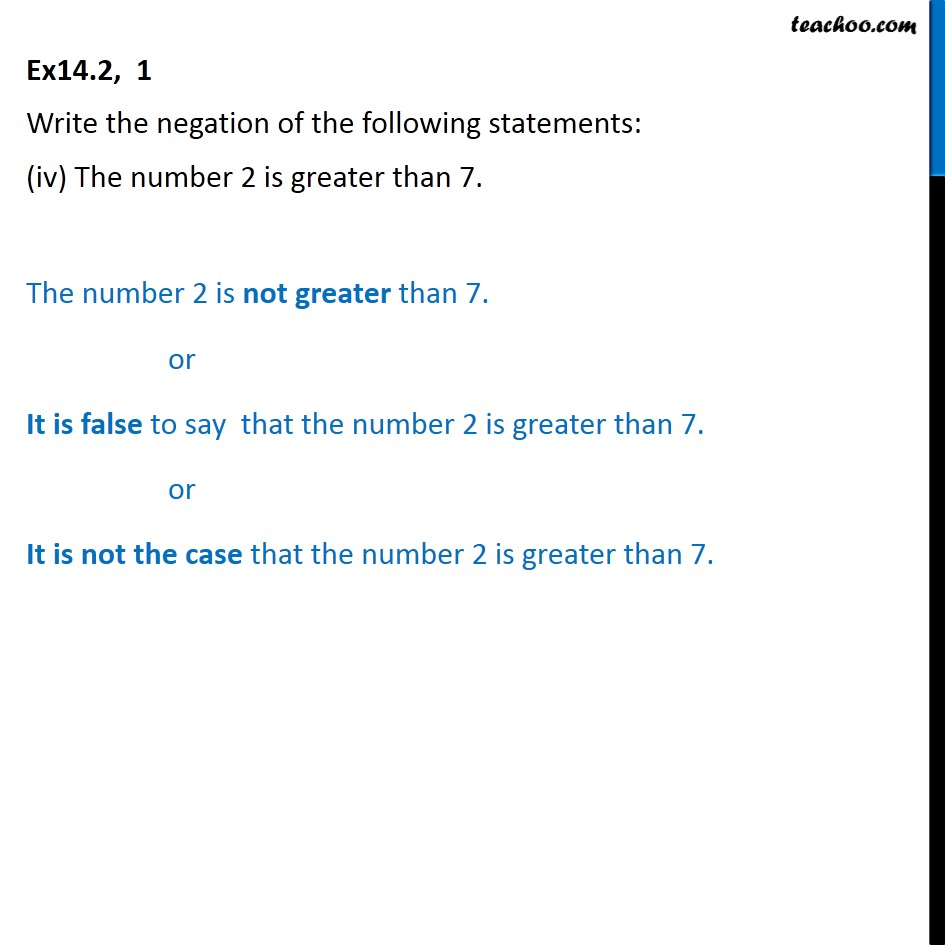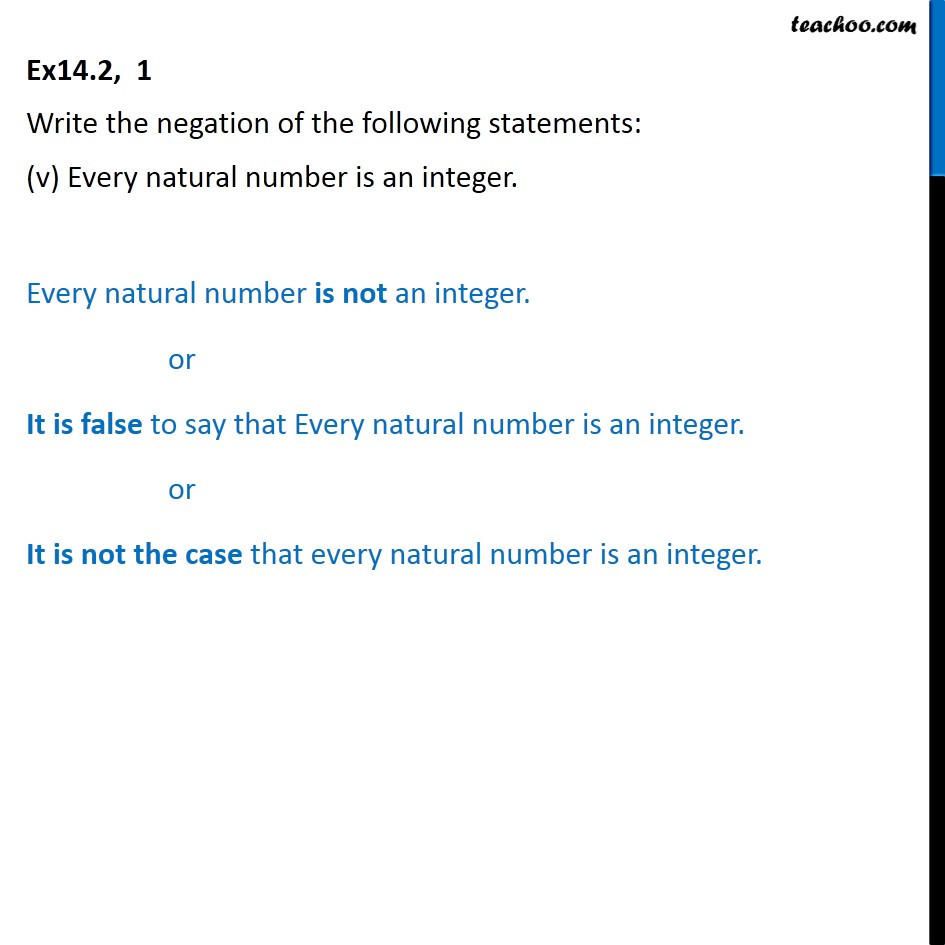1. Chapter 14 Class 11 Mathematical Reasoning
2. Serial order wise
3. Ex 14.2

Transcript

Ex 14.2, 1 Write the negation of the following statements: (i)Chennai is the capital of Tamil Nadu. Chennai is not the capital of Tamil Nadu. Or It is false to say that Chennai is the capital of Tamil Nadu. Or It is not the case that Chennai is the Capital of Tamil Nadu. Ex14.2, 1 Write the negation of the following statements: (ii) ﷐﷮2﷯ is not a complex number. ﷐﷮2﷯ is a complex number. or It is false to say that ﷐﷮2﷯ is not a Complex number or It is not the case that ﷐﷮2﷯ is not a complex number. Ex14.2, 1 Write the negation of the following statements: (iii) All triangles are not equilateral triangle. All triangles are equilateral triangle. or It is false to say that all triangles are not equilateral triangle. or It is not the case that all triangle are not equilateral triangle. Ex14.2, 1 Write the negation of the following statements: (iv) The number 2 is greater than 7. The number 2 is not greater than 7. or It is false to say that the number 2 is greater than 7. or It is not the case that the number 2 is greater than 7. Ex14.2, 1 Write the negation of the following statements: (v) Every natural number is an integer. Every natural number is not an integer. or It is false to say that Every natural number is an integer. or It is not the case that every natural number is an integer.

Ex 14.2